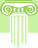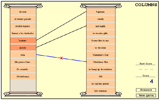Columns: Identify the matching terms in two columns.# Accounting for Plant Assets and Depreciation Quiz

Introduction of current assets and plant assets. Introduction of journalizing buying plant assets and posting the entry to the assets General ledger. Introduction of the effects of depreciation on plant assets, how to calculate the depreciation expense for an entire fiscal year, as well as part of a fiscal year using the Straight-Line Method of Depreciation. Introduction of journalizing and posting a plant assets depreciation expense and the sale of a plant asset.This activity was created by a Quia Web subscriber. Learn more about QuiaCreate your own activities